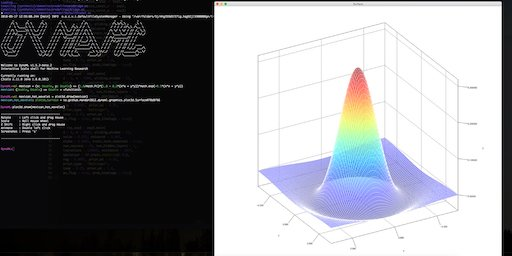# DynaML: ML + JVM + Scala¶

DynaML is a Scala & JVM Machine Learning toolbox for research, education & industry.## Motivation¶

• Interactive. Don't want to create Maven/sbt project skeletons every time you want to try out ideas? Create and execute scala worksheets in the DynaML shell. DynaML comes packaged with a customized version of the Ammonite REPL, with auto-complete, file operations and scripting capabilities.

• End to End. Create complex pre-processing pipelines with the data pipes API, train models (deep nets, gaussian processes, linear models and more), optimize over hyper-parameters, evaluate model predictions and visualise results.

• Enterprise Friendly. Take advantage of the JVM and Scala ecosystem, use Apache Spark to write scalable data analysis jobs, Tensorflow for deep learning, all in the same toolbox.

## Getting Started¶

### Platform Compatibility¶

Currently, only *nix and OSX platforms are supported.

DynaML is compatible with Scala 2.11

### Installation¶

Easiest way to install DynaML is cloning & compiling from the github repository. Please take a look at the installation instructions, to make sure that you have the pre-requisites and to configure your installation.

## CIFAR in 100 lines¶

Below is a sample script where we train a neural network of stacked Inception cells on the CIFAR-10 image classification task.

import ammonite.ops._
import io.github.mandar2812.dynaml.pipes.DataPipe
import io.github.mandar2812.dynaml.tensorflow.data.AbstractDataSet
import io.github.mandar2812.dynaml.tensorflow.{dtflearn, dtfutils}
import io.github.mandar2812.dynaml.tensorflow.implicits._
import org.platanios.tensorflow.api._
import org.platanios.tensorflow.api.learn.layers.Activation
import java.nio.file.Paths

val tempdir = home/"tmp"

Paths.get(tempdir.toString()),

val tf_dataset = AbstractDataSet(
dataSet.trainImages, dataSet.trainLabels, dataSet.trainLabels.shape(0),
dataSet.testImages, dataSet.testLabels, dataSet.testLabels.shape(0))

val trainData =
tf_dataset.training_data
.repeat()
.shuffle(10000)
.batch(128)
.prefetch(10)

println("Building the model.")
val input = tf.learn.Input(
UINT8,
Shape(
-1,
dataSet.trainImages.shape(1),
dataSet.trainImages.shape(2),
dataSet.trainImages.shape(3))
)

val trainInput = tf.learn.Input(UINT8, Shape(-1))

val relu_act = DataPipe[String, Activation](tf.learn.ReLU(_))

val architecture = tf.learn.Cast("Input/Cast", FLOAT32) >>
dtflearn.inception_unit(
channels = 3,  Seq.fill(4)(10),
relu_act)(layer_index = 1) >>
dtflearn.inception_unit(
channels = 40, Seq.fill(4)(5),
relu_act)(layer_index = 2) >>
tf.learn.Flatten("Layer_3/Flatten") >>
dtflearn.feedforward(256)(id = 4) >>
tf.learn.ReLU("Layer_4/ReLU", 0.1f) >>
dtflearn.feedforward(10)(id = 5)

val trainingInputLayer = tf.learn.Cast("TrainInput/Cast", INT64)

val loss =
tf.learn.SparseSoftmaxCrossEntropy("Loss/CrossEntropy") >>
tf.learn.Mean("Loss/Mean") >>
tf.learn.ScalarSummary("Loss/Summary", "Loss")

val optimizer = tf.train.Adam(0.1)

val summariesDir = Paths.get((tempdir/"cifar_summaries").toString())

val (model, estimator) = dtflearn.build_tf_model(
architecture, input, trainInput, trainingInputLayer,
loss, optimizer, summariesDir, dtflearn.max_iter_stop(500),
100, 100, 100)(
trainData, true)

def accuracy(predictions: Tensor, labels: Tensor): Float =
predictions.argmax(1)
.cast(UINT8)
.equal(labels)
.cast(FLOAT32)
.mean()
.scalar
.asInstanceOf[Float]

val (trainingPreds, testPreds): (Option[Tensor], Option[Tensor]) =
dtfutils.predict_data[
Tensor, Output, DataType, Shape, Output,
Tensor, Output, DataType, Shape, Output,
Tensor, Tensor](
estimator,
data = tf_dataset,
pred_flags = (true, true),
buff_size = 20000)

val (trainAccuracy, testAccuracy) = (
accuracy(trainingPreds.get, dataSet.trainLabels),
accuracy(testPreds.get, dataSet.testLabels))

print("Train accuracy = ")
pprint.pprintln(trainAccuracy)

print("Test accuracy = ")
pprint.pprintln(testAccuracy)